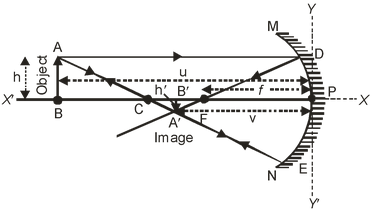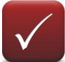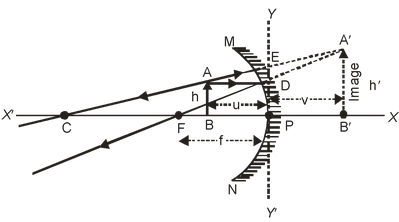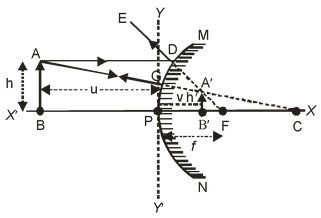# Some Important Conclusions

## On the basis of the New Cartesian Sign Convention discussed above, we can draw the following conclusions for the spherical mirrors (concave as well as convex mirrors).

### IN THE CASE OF CONCAVE MIRROR:

(i) For real and inverted image

 Focal length (f) = –ve Radius of curvature (R) = –ve Object distance (u) = –ve Object height (h) = +ve Image distance (v) = –ve Image height (h′) = –ve Magnification (m) = –veIn the case of a concave mirror, for real and inverted image, only object height (h) is +ve, and the remaining all distances are –ve.

(ii) For virtual and erect image

 Focal length (f) = –ve Radius of curvature (R) = –ve Object distance (u) = –ve Object height (h) = +ve Image distance (v) = +ve Image height (h′) = +ve Magnification (m) = +veIn the case of a concave mirror, for virtual and erect image, object distance (u) focal length (f), radius of curvature (R) are –ve and the remaining all distances are +ve.

### IN CASE OF CONVEX MIRROR

 A convex mirror always forms a virtual and erect image. Therefore for virtual and erect image: Focal length (f) = +ve Radius of curvature (R) = +ve Object distance (u) = –ve Object height (h) = +ve Image distance (v) = +ve Image height (h′) = +ve Magnification (m) = +veIn the case of a convex mirror, for virtual and erect image, only object distance (u) is –ve and the remaining all distances are +ve.

1. The radius of curvature of a spherical mirror is 20 cm. What is its focal length?

Solution: Focal length, f = Radiuns curvatures(R)/2

⇒ f = 20/2 = 10 cm.

2. Explain why, convex mirrors are used as rear-view mirrors in vehicles.

Solution: Convex mirrors are preferred as rear-view mirrors because of two reasons :

(a) A convex mirror always produces an erect (right side up) image of the objects.

(b) The image formed in a convex mirror is highly diminished or much smaller than the object, due to which a convex mirror gives a wide field of view (of the traffic behind). A convex mirror enables a driver to view much larger area of the traffic behind him than would be possible with a plane mirror. A plane mirror gives a narrow field of view.